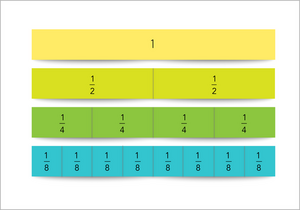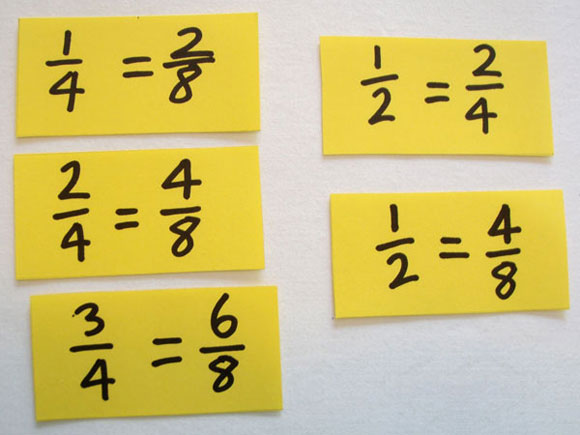# Fraction strips

Show an example of a fraction wall and explain that making a fraction wall will help to find equivalent fractions.

Working in small groups, students:

• cut a sheet of A3 or A4 paper longways into several strips
• establish that the length of the strip equals one whole
• fold each strip into a specified number of equal parts (e.g. 2, 4 and 8) keeping one strip whole
• label each of the parts with the unit fraction e.g. for the strip folded into four parts label each part as one-quarter (1/4).The strips are placed together so the size of the fractions can be compared.

Students record pairs of equivalent fractions, writing each pair onto a separate card.

Students look for similarities and sort the cards into groups. They describe any patterns they notice.

For example, in all the equivalent fractions for $$\frac{1}{2}$$ the denominator is multiplied by the same number as the numerator.Pairs of equivalent fractions.

Share the findings as a class and create a display of the fractions walls and equivalence cards.

Alternatively, each group could be invited to plan the set of fractions they want to represent.